Next: Interpretations of mutual information Up: content Previous: The stimulus set

## Calculating mutual information from experimental data: A primer

The mutual information (MI) between two random variables, such as stimuli S and neural responses R is defined in terms of their joint distribution. When this distribution is known exactly, the MI can be calculated as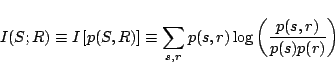(1)

where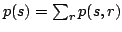andare the marginal distributions over the stimuli and responses respectively. Usually, neural responses are high dimensional and complex, and only some simplified version of the responsescan be considered. Examples for such simplifications are the total number of spikes in some window (spike count), the latency of the first spike after stimulus onset, or a coarse resolution binary pattern representation of the spiking activity.

Estimating MI from empirical data commonly involves two steps: first, estimating the joint distribution of stimuli and simplified responses, and then calculating the MI based on this estimated distribution. The first step in such calculations requires estimating the distribution of neural responses for each stimulus. For example, when interested in information in spike counts, one calculates the distribution of number of spikes in the responses, as measured across repeated presentation of each one of the stimuli separately. Repeating this calculation for each stimulus yields the joint distribution of stimuli and responses. An example of this procedure (using what is known as the maximum likelihood estimator) is given in Fig. 3 of the paper. Figure 3b shows raster plots of the responses to five different stimuli, and the number of spikes in each of thepresentations of the first stimulus is given in Table 1a below. The corresponding distribution of spike counts for the first stimulus is given in Table 1b below, and the distribution of spike counts for five representative stimuli is depicted in Fig. 3c. Figure 3d assembles all of these distributions together, forming the empirical joint distribution of stimuli and spike counts. Other statistics of spike patterns can be used instead of spike counts. For example, spike trains can be viewed as binary words'' of some fixed length, and their distribution can be estimated similarly to spike counts distribution by counting number of appearances of each word across stimulus repeated presentations (Fig 3e).

a.
 trial no 1 2 3 4 5 6 7 8 9 10 # spikes 6 6 6 6 5 6 5 7 3 6 trial no 11 12 13 14 15 16 17 18 19 20 # spikes 5 4 4 5 6 7 6 6 9 5

b.
 # spikes 1 2 3 4 5 6 7 8 9 10 probability 0 0 0.05 0.1 0.25 0.45 0.1 0 0.05 0
Table 1: Maximum likelihood estimation of spike count distribution. (a) The number of spikes in each of 20 stimulus presentations. (b) The resulting estimated distribution.

The second step is to calculate MI from the joint distribution. When the number of samples is very large relative to the number of bins in the joint distribution matrix, the observed empirical joint distributionprovides a good estimate of the true underlying distribution, and the MI can be calculated by plugging in the empirical distribution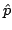into the MI formula ,(2)

whereand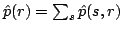are the marginal empirical distributions over the stimuli and responses respectively. Unfortunately, with common experimental settings the number of samples is often not sufficient, and this naive MI estimator is positively biased: it tends to produce overestimates of the MI relative to the MI of the true distribution,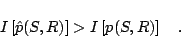(3)

In addition, the variability of the estimator due to finite sampling is also considerable. It has been shown that a first order approximation of the bias is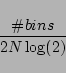(4)

where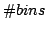is the number of degrees of freedom and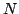is the number of samples [4,6]. Subtracting this estimate of the bias from the empirical MI estimate reduces substantially the bias.

Since the bias is roughly proportional to the number of bins in the joint distribution matrix, we have performed a procedure that iteratively unites rows or columns of the matrix. At each step, the row or column with minimum marginal probability was united with its neighbour with the lower marginal probability. The MI was determined as the largest bias-corrected estimate among all tested reduced matrices. This matrix reduction reduces the information in the matrix, but at the same time reduces the bias, and therefore makes it possible to obtain higher and more reliable estimates of the MI. The performance of this algorithm was discussed in detail in .Next: Interpretations of mutual information Up: suppl_html Previous: The stimulus set
Gal Chechik 2006-07-19